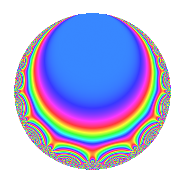# Properties

 Label 189.2.e.dLevel 189 Weight 2 Character orbit 189.e Analytic conductor 1.509 Analytic rank 0 Dimension 4 CM No Inner twists 4

# Learn more about

## Newspace parameters

 Level: $$N$$ = $$189 = 3^{3} \cdot 7$$ Weight: $$k$$ = $$2$$ Character orbit: $$[\chi]$$ = 189.e (of order $$3$$ and degree $$2$$)

## Newform invariants

 Self dual: No Analytic conductor: $$1.5091725982$$ Analytic rank: $$0$$ Dimension: $$4$$ Relative dimension: $$2$$ over $$\Q(\zeta_{3})$$ Coefficient field: $$\Q(\sqrt{-2}, \sqrt{-3})$$ Coefficient ring: $$\Z[a_1, \ldots, a_{7}]$$ Coefficient ring index: $$3$$ Sato-Tate group: $\mathrm{SU}(2)[C_{3}]$

## $q$-expansion

Coefficients of the $$q$$-expansion are expressed in terms of a basis $$1,\beta_1,\beta_2,\beta_3$$ for the coefficient ring described below. We also show the integral $$q$$-expansion of the trace form.

 $$f(q)$$ $$=$$ $$q$$ $$-\beta_{2} q^{2}$$ $$+ ( -4 + 4 \beta_{1} ) q^{4}$$ $$-\beta_{2} q^{5}$$ $$+ ( 1 - 3 \beta_{1} ) q^{7}$$ $$+ 2 \beta_{3} q^{8}$$ $$+O(q^{10})$$ $$q$$ $$-\beta_{2} q^{2}$$ $$+ ( -4 + 4 \beta_{1} ) q^{4}$$ $$-\beta_{2} q^{5}$$ $$+ ( 1 - 3 \beta_{1} ) q^{7}$$ $$+ 2 \beta_{3} q^{8}$$ $$+ ( -6 + 6 \beta_{1} ) q^{10}$$ $$+ ( 2 \beta_{2} - 2 \beta_{3} ) q^{11}$$ $$-4 q^{13}$$ $$+ ( 2 \beta_{2} - 3 \beta_{3} ) q^{14}$$ $$-4 \beta_{1} q^{16}$$ $$+ ( -\beta_{2} + \beta_{3} ) q^{17}$$ $$+ \beta_{1} q^{19}$$ $$+ 4 \beta_{3} q^{20}$$ $$+ 12 q^{22}$$ $$-\beta_{2} q^{23}$$ $$+ ( -1 + \beta_{1} ) q^{25}$$ $$+ 4 \beta_{2} q^{26}$$ $$+ ( 8 + 4 \beta_{1} ) q^{28}$$ $$-3 \beta_{3} q^{29}$$ $$+ ( 7 - 7 \beta_{1} ) q^{31}$$ $$-6 q^{34}$$ $$+ ( 2 \beta_{2} - 3 \beta_{3} ) q^{35}$$ $$-8 \beta_{1} q^{37}$$ $$+ ( -\beta_{2} + \beta_{3} ) q^{38}$$ $$-12 \beta_{1} q^{40}$$ $$+ 3 \beta_{3} q^{41}$$ $$- q^{43}$$ $$-8 \beta_{2} q^{44}$$ $$+ ( -6 + 6 \beta_{1} ) q^{46}$$ $$-\beta_{2} q^{47}$$ $$+ ( -8 + 3 \beta_{1} ) q^{49}$$ $$+ \beta_{3} q^{50}$$ $$+ ( 16 - 16 \beta_{1} ) q^{52}$$ $$+ ( -\beta_{2} + \beta_{3} ) q^{53}$$ $$+ 12 q^{55}$$ $$+ ( -6 \beta_{2} + 2 \beta_{3} ) q^{56}$$ $$+ 18 \beta_{1} q^{58}$$ $$+ ( -4 \beta_{2} + 4 \beta_{3} ) q^{59}$$ $$-5 \beta_{1} q^{61}$$ $$-7 \beta_{3} q^{62}$$ $$-8 q^{64}$$ $$+ 4 \beta_{2} q^{65}$$ $$+ ( -2 + 2 \beta_{1} ) q^{67}$$ $$+ 4 \beta_{2} q^{68}$$ $$+ ( 12 + 6 \beta_{1} ) q^{70}$$ $$+ ( 1 - \beta_{1} ) q^{73}$$ $$+ ( 8 \beta_{2} - 8 \beta_{3} ) q^{74}$$ $$-4 q^{76}$$ $$+ ( 2 \beta_{2} + 4 \beta_{3} ) q^{77}$$ $$+ 4 \beta_{1} q^{79}$$ $$+ ( 4 \beta_{2} - 4 \beta_{3} ) q^{80}$$ $$-18 \beta_{1} q^{82}$$ $$+ 6 \beta_{3} q^{83}$$ $$-6 q^{85}$$ $$+ \beta_{2} q^{86}$$ $$+ ( -24 + 24 \beta_{1} ) q^{88}$$ $$-\beta_{2} q^{89}$$ $$+ ( -4 + 12 \beta_{1} ) q^{91}$$ $$+ 4 \beta_{3} q^{92}$$ $$+ ( -6 + 6 \beta_{1} ) q^{94}$$ $$+ ( -\beta_{2} + \beta_{3} ) q^{95}$$ $$- q^{97}$$ $$+ ( 5 \beta_{2} + 3 \beta_{3} ) q^{98}$$ $$+O(q^{100})$$ $$\operatorname{Tr}(f)(q)$$ $$=$$ $$4q$$ $$\mathstrut -\mathstrut 8q^{4}$$ $$\mathstrut -\mathstrut 2q^{7}$$ $$\mathstrut +\mathstrut O(q^{10})$$ $$4q$$ $$\mathstrut -\mathstrut 8q^{4}$$ $$\mathstrut -\mathstrut 2q^{7}$$ $$\mathstrut -\mathstrut 12q^{10}$$ $$\mathstrut -\mathstrut 16q^{13}$$ $$\mathstrut -\mathstrut 8q^{16}$$ $$\mathstrut +\mathstrut 2q^{19}$$ $$\mathstrut +\mathstrut 48q^{22}$$ $$\mathstrut -\mathstrut 2q^{25}$$ $$\mathstrut +\mathstrut 40q^{28}$$ $$\mathstrut +\mathstrut 14q^{31}$$ $$\mathstrut -\mathstrut 24q^{34}$$ $$\mathstrut -\mathstrut 16q^{37}$$ $$\mathstrut -\mathstrut 24q^{40}$$ $$\mathstrut -\mathstrut 4q^{43}$$ $$\mathstrut -\mathstrut 12q^{46}$$ $$\mathstrut -\mathstrut 26q^{49}$$ $$\mathstrut +\mathstrut 32q^{52}$$ $$\mathstrut +\mathstrut 48q^{55}$$ $$\mathstrut +\mathstrut 36q^{58}$$ $$\mathstrut -\mathstrut 10q^{61}$$ $$\mathstrut -\mathstrut 32q^{64}$$ $$\mathstrut -\mathstrut 4q^{67}$$ $$\mathstrut +\mathstrut 60q^{70}$$ $$\mathstrut +\mathstrut 2q^{73}$$ $$\mathstrut -\mathstrut 16q^{76}$$ $$\mathstrut +\mathstrut 8q^{79}$$ $$\mathstrut -\mathstrut 36q^{82}$$ $$\mathstrut -\mathstrut 24q^{85}$$ $$\mathstrut -\mathstrut 48q^{88}$$ $$\mathstrut +\mathstrut 8q^{91}$$ $$\mathstrut -\mathstrut 12q^{94}$$ $$\mathstrut -\mathstrut 4q^{97}$$ $$\mathstrut +\mathstrut O(q^{100})$$

Basis of coefficient ring in terms of a root $$\nu$$ of $$x^{4}\mathstrut -\mathstrut$$ $$2$$ $$x^{2}\mathstrut +\mathstrut$$ $$4$$:

 $$\beta_{0}$$ $$=$$ $$1$$ $$\beta_{1}$$ $$=$$ $$\nu^{2}$$$$/2$$ $$\beta_{2}$$ $$=$$ $$($$$$\nu^{3} + 2 \nu$$$$)/2$$ $$\beta_{3}$$ $$=$$ $$($$$$-\nu^{3} + 4 \nu$$$$)/2$$
 $$1$$ $$=$$ $$\beta_0$$ $$\nu$$ $$=$$ $$($$$$\beta_{3}\mathstrut +\mathstrut$$ $$\beta_{2}$$$$)/3$$ $$\nu^{2}$$ $$=$$ $$2$$ $$\beta_{1}$$ $$\nu^{3}$$ $$=$$ $$($$$$-$$$$2$$ $$\beta_{3}\mathstrut +\mathstrut$$ $$4$$ $$\beta_{2}$$$$)/3$$

## Character Values

We give the values of $$\chi$$ on generators for $$\left(\mathbb{Z}/189\mathbb{Z}\right)^\times$$.

 $$n$$ $$29$$ $$136$$ $$\chi(n)$$ $$1$$ $$-\beta_{1}$$

## Embeddings

For each embedding $$\iota_m$$ of the coefficient field, the values $$\iota_m(a_n)$$ are shown below.

For more information on an embedded modular form you can click on its label.

Label $$\iota_m(\nu)$$ $$a_{2}$$ $$a_{3}$$ $$a_{4}$$ $$a_{5}$$ $$a_{6}$$ $$a_{7}$$ $$a_{8}$$ $$a_{9}$$ $$a_{10}$$
109.1
 1.22474 + 0.707107i −1.22474 − 0.707107i 1.22474 − 0.707107i −1.22474 + 0.707107i
−1.22474 2.12132i 0 −2.00000 + 3.46410i −1.22474 2.12132i 0 −0.500000 2.59808i 4.89898 0 −3.00000 + 5.19615i
109.2 1.22474 + 2.12132i 0 −2.00000 + 3.46410i 1.22474 + 2.12132i 0 −0.500000 2.59808i −4.89898 0 −3.00000 + 5.19615i
163.1 −1.22474 + 2.12132i 0 −2.00000 3.46410i −1.22474 + 2.12132i 0 −0.500000 + 2.59808i 4.89898 0 −3.00000 5.19615i
163.2 1.22474 2.12132i 0 −2.00000 3.46410i 1.22474 2.12132i 0 −0.500000 + 2.59808i −4.89898 0 −3.00000 5.19615i
 $$n$$: e.g. 2-40 or 990-1000 Significant digits: Format: Complex embeddings Normalized embeddings Satake parameters Satake angles

## Inner twists

Char. orbit Parity Mult. Self Twist Proved
1.a Even 1 trivial yes
3.b Odd 1 yes
7.c Even 1 yes
21.h Odd 1 yes

## Hecke kernels

This newform can be constructed as the kernel of the linear operator $$T_{2}^{4}$$ $$\mathstrut +\mathstrut 6 T_{2}^{2}$$ $$\mathstrut +\mathstrut 36$$ acting on $$S_{2}^{\mathrm{new}}(189, [\chi])$$.